# Math in Focus Grade 3 Cumulative Review Chapters 10 to 12 Answer Key

Practice the problems of Math in Focus Grade 3 Workbook Answer Key Cumulative Review Chapters 10 to 12 to score better marks in the exam.

## Math in Focus Grade 3 Cumulative Review Chapters 10 to 12 Answer Key

Concepts and Skills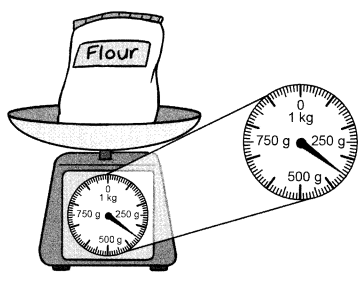Question 1.
The mass of the bag of flour is ___________ grams.
The mass of the bag of flour is 350 grams.
Explanation:
According to the measure in the scale the scale shows 350

Write true or false.

Question 2.
6,016 meters is the same as 6 meters 16 centimeters. (Lesson 11.1)
yes, both are same
Explanation:
1 meter = 1000 centimeters
6 x 1000 = 6000
6000 + 16 = 6016 centimeters.

Question 3.
7 kilograms 3 grams is the same as 7,030 grams. (Lesson 11.3)
Yes, both are same.
Explanation:
1 Kilogram = 1000 grams
7 x 1000 = 7000 grams
7000 + 30 = 7030 grams

Question 4.
4 liters 250 milliliters is the same as 4,250 milliliters. (Lesson 11.4)
Yes, Both are same
Explanation:
1 liter = 1000 milliliters
4 x 1000 = 4000 milliliters
4000 + 250 = 4250 milliliters

Fill in the blanks.

The table shows the height of five students. (Lesson 11.1)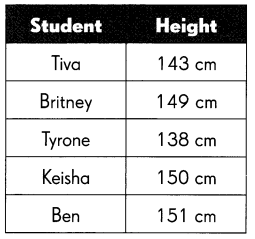Question 5.
Britney is ___________ meter ___________ centimeters tall.
1 meter = 100 centimeter
1.49 meters
Britney is 1.49 meter 149 centimeters tall.

Question 6.
The height of the tallest student is ___________ meter ___________ centimeters.
151 centimeters
1 meter = 100 centimeter
1.51 meters
The height of the tallest student is 1.51 meter 151 centimeters.

Question 7.
Keisha is ___________ centimeters taller than Tyrone.
Keisha = 150
and  Tyrone = 138
150 – 138 = 12
Keisha is 12 centimeters taller than Tyrone.

Question 8.
Which bag has a different mass from the others? (Lessor 11.3)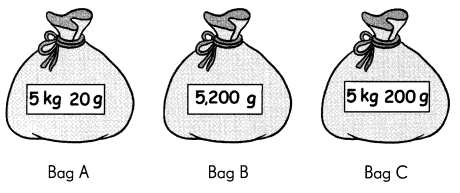Bag __________ has a different mass from the other two.
Bag  C has a different mass from the other two.
Explanation:
Bag A and Bag B has same weights
1 kg = 1000 g
5 kg 20 g = 5200 g

Fill in the blanks. (Lesson 11.4)

Each container is completely filled with water. The water is emptied into measuring cups.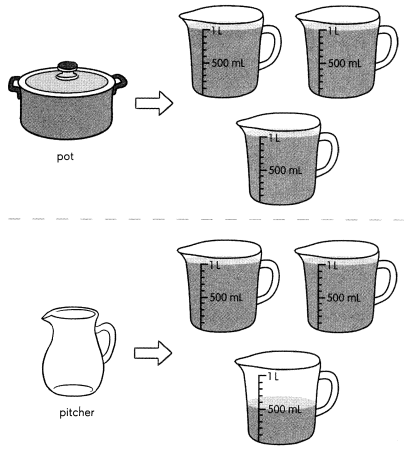Question 9.
The ___________ has the greater capacity.
The pot has the greater capacity.
Explanation:
As the pot can contain 3 liters
and the pitcher has 2 liters 250
2250 liters.

Question 10.
The container with the greater capacity can hold ____________ milliliters of water.
3 x 1 = 3 liters
1 liter = 1000 milliliters
Explanation:
The container with the greater capacity can hold 3000 milliliters of water.

Look at the picture. Complete. (Lesson 11.2)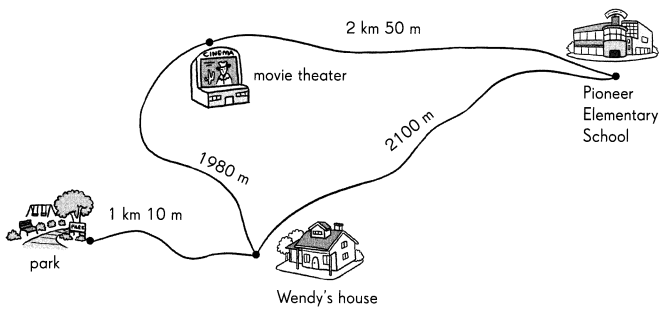Question 11.
The distance between Wendy’s house and Pioneer Elementary School is ____________ kilometers ____________ meters.
The distance between Wendy’s house and Pioneer Elementary School is 2 kilometers 100 meters.
Explanation:
1 kilometer = 1000 meters
So 2100 meters = 2000 meters + 100 meters = 2 kilometers and 100 meters

Question 12.
The distance between the movie theater and the Pioneer Elementary School is ____________ meters.
The distance between the movie theater and the Pioneer Elementary School is 2050 meters.
Explanation:
1 kilometer = 1000 meters
So 2km 50 meters = 2000 meters + 50 meters = 2050 meters.

Question 13.
The distance between the park and Wendy’s house is ____________ meters.
The distance between the park and Wendy’s house is 1010 meters.
Explanation:
1 kilometer = 1000 meters
So 1km 10 meters = 1000 meters + 10 meters = 1010  meters.

Question 14.
The distance between Wendy’s house and the movie theater is ____________ kilometer ____________ meters.
The distance between Wendy’s house and the movie theater is 1 kilometer 980 meters.
Explanation:
1 kilometer = 1000 meters
So 1980meters = 1000 meters + 980 meters = 1 kilometer and 980 meters

Question 12.

Problem Solving

Question 15.
Hayley is saving for a book that costs $19.50. She has saved$7.85. How much more does she need to save?
Answer:$11.65 Explanation: Hayley is saving for a book that costs$19.50. She has saved $7.85.$11.65 more she need to save.
Since $19.50 –$7.85= $11.65 Question 16. The table shows the amount of money Tim saved for three months.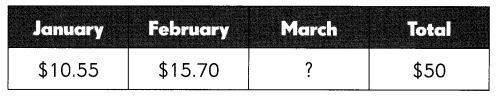How much did Tim save in March?$ ____________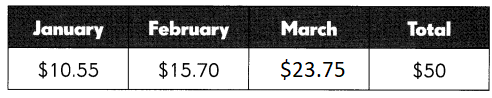How much did Tim save in March? $23.75 Explanation: Jan and Feb total savings are$10.55 + $15.70 =$26.25
Total Jan, Feb ad Mar savings are  $50. So March month savings are$50 – $26.25 =$23.75

Question 17.
Nigel has 1 3 quarters. Madi has $6.85. How much money do they have in all? Answer:$6.98
Explanation:
Nigel has 1 3 quarters. Madi has $6.85. As they are quarters we have to add in decimal point. 6.85 + 13 = 6.98 Question 18. The mass of 3 tomatoes and a squash is 730 grams. The mass of the squash is 430 grams. What is the mass of one tomato? Answer: the mass of one tomato = 100 grams Explanation: The mass of 3 tomatoes and a squash is 730 grams. The mass of the squash is 430 grams. 730 – 430 = 300 300 ÷ 3 = 100 Solve. Show your work. Question 19. A sparrow flies 256 meters. An eagle flies three times as far as the sparrow. The distance flown by the eagle is twice that of the pigeon. How far does the pigeon fly? Answer: 1536 meters Explanation: A sparrow flies 256 meters. An eagle flies three times as far as the sparrow. 3 x 256 = 768 The distance flown by the eagle is twice that of the pigeon. 768 x 2 = 1536 1536 meters that the pigeon fly Question 20. An equal amount of lemonade is poured into 3 cups. The pitcher has 40 milliliters left in it. Each cup has 325 milliliters of lemonade. How much lemonade was in the pitcher at first? Express your answer in liters and milliliters. Answer: 1050 in milliliters 1.050 in liters Explanation: An equal amount of lemonade is poured into 3 cups. The pitcher has 40 milliliters left in it. Each cup has 325 milliliters of lemonade. 325 x 3 = 975 975 + 40 = 1050 1050 milliliters lemonade was in the pitcher at first 1 liter = 1000 milliliter Solve. Show your work. Question 21. Elle had$85.30. After buying two of these items, she has $25.75 left. a. How much did the two items cost? Answer:$59.55 was the item cost
Explanation:
Elle had $85.30. After buying two of these items, she has$25.75 left.
$85.30 – 25.75 =$59.55

b. Which two items did she buy? Do you need an exact answer or an estimate to solve this?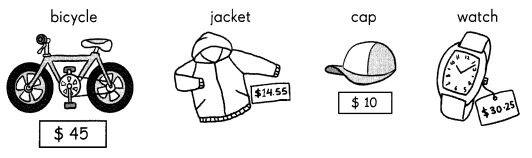$45 +$15.55 = \$59.55